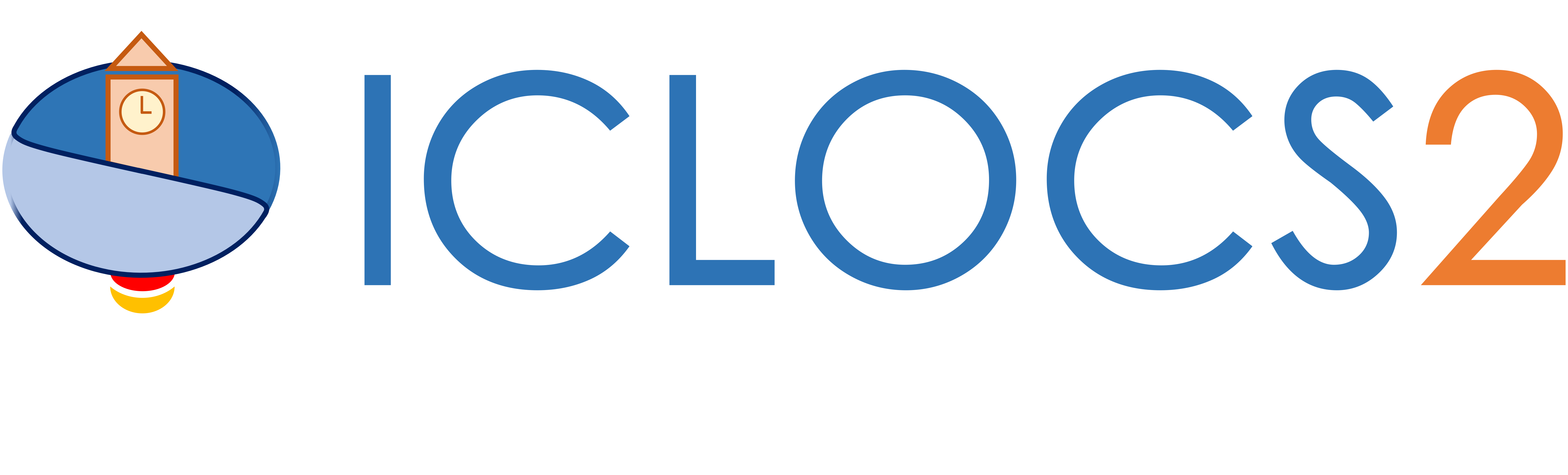Example: Control of a Spacecraft

Difficulty: Intermediate

This problem was originally presented in , and this implementation was adapted from . This example is available via the link on the right.

The implementation of this examples will not be explained in details due to similarity with other examples. Please refer to an example with similar complexity for implementation instructions, or other examples in the list of examples with Detailed Instructions.

Results from ICLOCS2

Using the Hermite-Simpson discretization scheme of ICLOCS2, the following state and input trajectories are obtained under a mesh refinement scheme starting with 20 mesh points. The computation time with IPOPT (NLP convergence tol set to 1e-09) is about 0.7 seconds, using finite difference derivative calculations on an Intel i7-6700 desktop computer.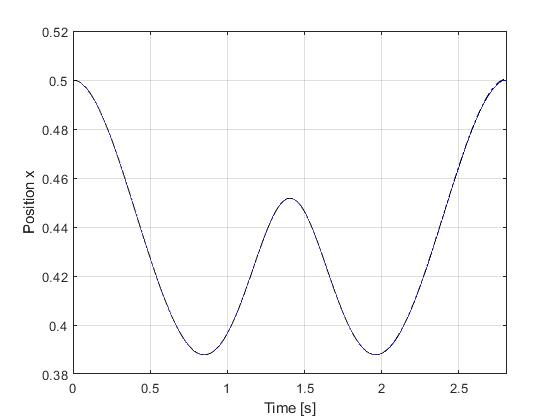Figure 1: Optimal state (x position) trajectory for the control of a spacecraft problem (dash black line indicate the open-loop simulation of the obtained solution)Figure 2: Optimal state (y position) trajectory for the control of a spacecraft problem (dash black line indicate the open-loop simulation of the obtained solution)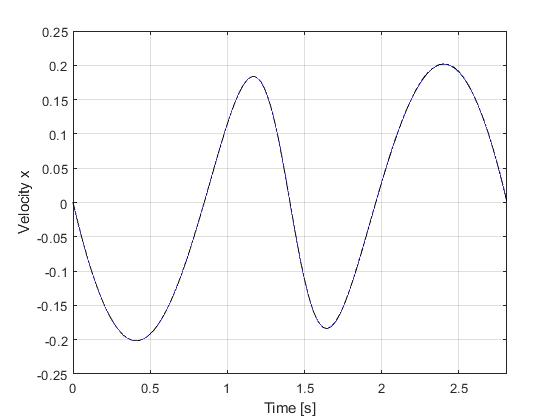Figure 3: Optimal state (x velocity) trajectory for the control of a spacecraft problem (dash black line indicate the open-loop simulation of the obtained solution)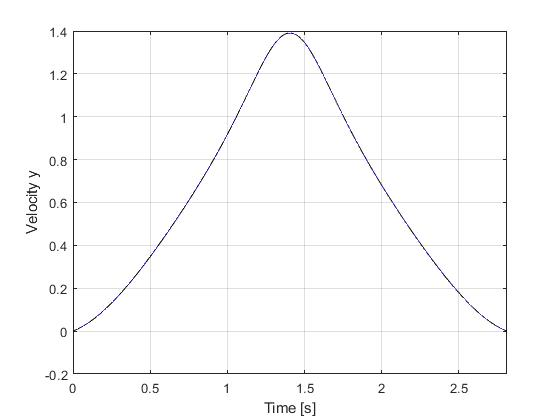Figure 4: Optimal state (y velocity) trajectory for the control of a spacecraft problem (dash black line indicate the open-loop simulation of the obtained solution)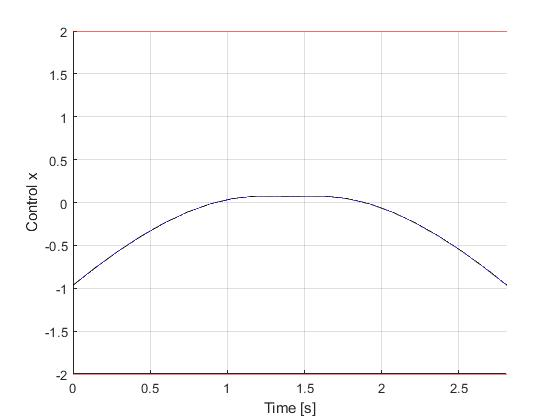Figure 5: Optimal input (u_x) trajectory for the control of a spacecraft problem (dash black line indicate the open-loop simulation of the obtained solution)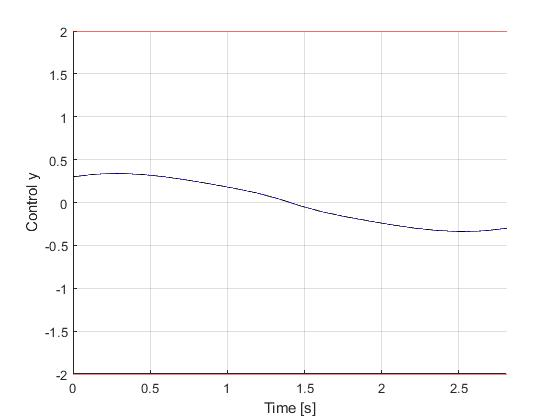Figure 6: Optimal input (u_y) trajectory for the control of a spacecraft problem (dash black line indicate the open-loop simulation of the obtained solution)

 Battin, R.H.: An Introduction to the Mathematics and Methods of Astrodynamics, Revised Edition. AIAA Education Series (1999)

 Knauer M., Buekens C. (2019) Real-Time Optimal Control Using TransWORHP and WORHP Zen. In: Fasano G., Pinter J. (eds) Modeling and Optimization in Space Engineering. Springer Optimization and Its Applications, vol 144. Springer, Cham